International
Tables for
Crystallography
Volume B
Reciprocal space
Edited by U. Shmueli

International Tables for Crystallography (2006). Vol. B. ch. 2.5, p. 317   | 1 | 2 |

## Section 2.5.6.3. Discretization

B. K. Vainshteinc

#### 2.5.6.3. Discretization

| top | pdf |

In direct methods of reconstruction as well as in Fourier methods the space is represented as a discrete set of points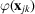on a two-dimensional net or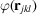on a three-dimensional lattice. It is sometimes expedient to use cylindrical or spherical coordinates. In two-dimensional reconstruction the one-dimensional projections are represented as a set of discrete values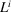, at a certain spacing in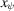. The reconstruction (2.5.6.9)is carried out over the discrete net with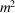nodes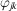. The net side A should exceed the diameter of an object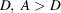; the spacing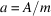. Then (2.5.6.8)transforms into the sum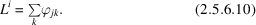For oblique projections the above sum is taken over all the points within the strips of width a along the axis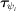(Fig. 2.5.6.4).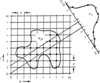Figure 2.5.6.4 | top | pdf |Discretization and oblique projection.

The resolution δ of the reconstructed function depends on the number h of the available projections. At approximately uniform angular distribution of projections, and diameter equal to D, the resolution at reconstruction is estimated as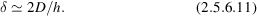The reconstruction resolution δ should be equal to or somewhat better than the instrumental resolution d of electron micrographs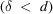, the real resolution of the reconstructed structure being d. If the number of projections h is not sufficient, i.e.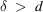, then the resolution of the reconstructed structure is δ (Crowther, DeRosier & Klug, 1970; Vainshtein, 1978).

In electron microscopy the typical instrumental resolution d of biological macromolecules for stained specimens is about 20 Å; at the object with diameter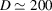Å the sufficient number h of projections is about 20. If the projections are not uniformly distributed in projection angles, the resolution decreases towards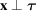for such τ in which the number of projections is small.

Properties of projections of symmetric objects . If the object has an N-fold axis of rotation, its projection has the same symmetry. At orthoaxial projection perpendicular to the N-fold axis the projections which differ in angle at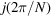are identical: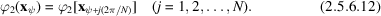This means that one of its projections is equivalent to N projections. If we have h independent projections of such a structure, the real number of projections is hN (Vainshtein, 1978). For a structure with cylindrical symmetry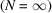one of its projections fully determines the three-dimensional structure.

Many biological objects possess helical symmetry – they transform into themselves by the screw displacement operation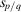, where p is the number of packing units in the helical structure per q turns of the continuous helix. In addition, the helical structures may also have the axis of symmetry N defining the pitch of the helix. In this case, a single projection is equivalent to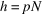projections (Cochran et al., 1952).

Individual protein molecules are described by point groups of symmetry of type N or. Spherical viruses have icosahedral symmetry 532 with two-, three- and fivefold axes of symmetry. The relationship between vectors τ of projections is determined by the transformation matrix of the corresponding point group (Crowther, Amos et al., 1970).

### ReferencesCochran, W., Crick, F. H. C. & Vand, V. (1952). The structure of synthetic polypeptides. 1. The transform of atoms on a helix. Acta Cryst. 5, 581–586.Google ScholarCrowther, R. A., Amos, L. A., Finch, J. T., DeRosier, D. J. & Klug, A. (1970). Three dimensional reconstruction of spherical viruses by Fourier synthesis from electron micrographs. Nature (London), 226, 421–425.Google ScholarCrowther, R. A., DeRosier, D. J. & Klug, A. (1970). The reconstruction of a three-dimensional structure from projections and its application to electron microscopy. Proc. R. Soc. London Ser. A, 317, 319–340.Google ScholarVainshtein, B. K. (1978). Electron microscopical analysis of the three-dimensional structure of biological macromolecules. In Advances in optical and electron microscopy, Vol. 7, edited by V. E. Cosslett & R. Barer, pp. 281–377. London: Academic Press.Google Scholar